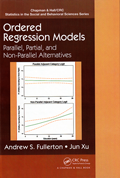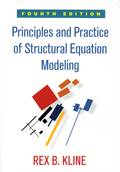Home  /  Stata News  /  Vol 31 No 2 (2016 quarter 2)  /  Bookstore## Biostatistics in Public Health Using StataThis book demonstrates the use of Stata for statistical analyses common in public health research and in many other disciplines. For those new to Stata, the authors first provide an introduction to Stata’s interface and to commands for descriptive statistics and graphics. In the discussions of statistical procedures that follow, readers will find extensive coverage of Stata’s menus, dialog boxes, commands, and output. Topics include linear regression, analysis of variance, logistic regression, Poisson regression, survival analysis, multilevel mixed-effects models, and power and sample-size analysis.

## Ordered Regression Models: Parallel, Partial, and Non-Parallel AlternativesIn Ordered Regression Models: Parallel, Partial, and Non-Parallel Alternatives, Fullerton and Xu provide a thorough treatment of models for ordinal data. This book will appeal to researchers from any discipline who wish to build on their knowledge of linear, logistic, and probit regression and learn both theoretical and practical concepts related to a variety of models for ordinal outcomes.

As the title indicates, the models presented are partitioned into three groups based on whether a parallel regression assumption is made for all covariates, for a subset of the covariates, or for none of the covariates. Under each of these assumptions, the authors describe three models—cumulative, continuation ratio, and adjacent category—from which a researcher can choose, depending on the probability of interest. They also include worked examples with real data and provide advice regarding interpretation, presentation of results, choice of model, and common problems that arise. Example Stata commands for fitting these models are shown at the end of each chapter.

## Principles and Practice of Structural Equation Modeling, Fourth EditionThe fourth edition of Principles and Practice of Structural Equation Modeling by Rex Kline, like previous editions, is an ideal text for both students and researchers who want to learn the fundamental concepts of structural equation modeling (SEM) and then apply it to their own data. Along with introducing different types of structural equation models, Kline carefully discusses practical issues, such as data preparation, assumptions, identification, and interpretation. Easy-to-follow examples use real data, and the book's website provides files demonstrating how to reproduce results using a variety of software packages, including Stata.

In the fourth edition, Kline adds new coverage of Judea Pearl's structural causal modeling, confirmatory factor analysis with ordinal indicators, bootstrapping, significance testing, and item response theory.

Browse these and other titles in our Bookstore.